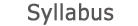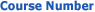0321-1839 Introductory Mathematics for Physicists 22019 - 2020View groups
University credit hours:  6.0

Course description

Introductory Mathematics for Physicists 2 Syllabus

Instructor

Rennan Barkana

Email: barkana@tau.ac.il

Spring semester

Number of Hours/ Credits

4 lecture + 2 rec.

Mandatory/Elective

Mandatory

Prerequisites

Introductory Mathematics for Physicists 1, Calculus 1A for Physicists

Year in program & how often given, if relevant

Year 1, given every year

Course overview – short abstract

This course gives first-year physics students further mathematical technical skills that are necessary for the physics courses being taken during their first and second years. Material includes linear algebra (skipped by students who learn this in Math or other departments), vector analysis, and an introduction to tensor analysis.

Learning outcomes – short description (if you don’t have LOs, then don’t write anything in this part)

Assignments – 10%

Final exam – 90%

Week 1: Parametric curves, basics of scalar and vector fields

Week 2: Line, area, and volume integrals

Week 4: Gauss, Stokes, Green theorems

Week 5: Vectors in general coordinate systems

Week 6: Linear algebra: Linear equations, Gauss-Jordan elimination

Week 7: Sets, groups

Week 8: Fields, Vector spaces

Week 9: Basis of a vector space, Matrices

Week 10: Inner and outer product, Gram-Schmidt orthogonalization

Week 11: Linear mappings, similarity transformations

Week 12: Eigenvalues, diagonalization of matrices

Week 13: Intro to Tensor analysis

Required text: None

accessibility declaration